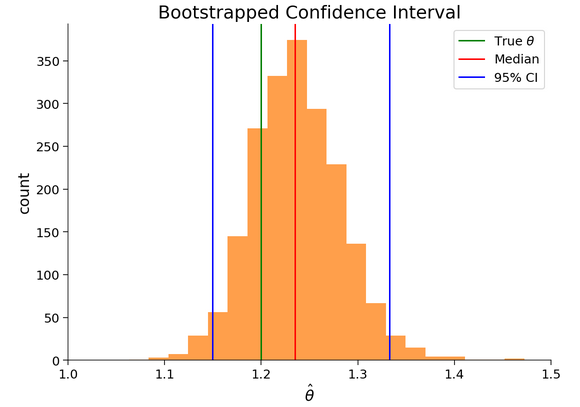# W1D3 Tutorial 3: Bootstrapping Regression Coefficients

In Tutorial 3 Section 2, where we try to determine a 95% confidence interval for the bootstrapped estimate of theta, the underlying assumption is that the distribution of these bootstrapped estimates is normal, or normal-like, or at reasonably non-skewed, uni-modal. Will this distribution always have these characteristics? What prevents it from being bi-modal or something else entirely?1 Like

I don’t think we are assuming that the estimates are normal here. The confidence interval is estimated directly from the bootstrap samples by calculating percentiles; z-score and (inverse) cumulative normal distribution functions are not used in this case.

1 Like